6224.0.55.001 - Labour Force, Australia: Labour Force Status and Other Characteristics of Families, June 2017
Previous ISSUE Released at 11:30 AM (CANBERRA TIME) 04/12/2017
 Page tools: .mffeedback,#pjs { display :none; } Enable Javascript to Print PagesPrint AllEmail NotificationRSSSearch this Product Explanatory Notes Glossary Quality Declaration - Summary Data Quality (Technical Note) (Technical Note) INTRODUCTION 1 Since the estimates in this publication are based on information obtained from occupants of a sample of households, they are subject to sampling variability. That is, they may differ from those estimates that would have been produced if all households had been included in the survey or a different sample was selected. One measure of the likely difference is given by the standard error (SE), which indicates the extent to which an estimate might have varied by chance because only a sample of households was included. There are about two chances in three (67%) that a sample estimate will differ by less than one SE from the number that would have been obtained if all households had been included, and about 19 chances in 20 (95%) that the difference will be less than two SEs. 2 Another measure of the likely difference is the relative standard error (RSE), which is obtained by expressing the SE as a percentage of the estimate. RSE% = (SE/estimate ) x 100 3 RSEs for Labour Force Status and Other Characteristics of Families estimates have been calculated using the Jackknife method of variance estimation. This process involves the calculation of 30 'replicate' estimates based on 30 different sub-samples of the original sample. The variability of estimates obtained from these sub-samples is used to estimate the sample variability surrounding the main estimate. 4 The Excel spreadsheets in the Downloads tab contain all the tables produced for this release and the calculated RSEs for each of the estimates. The RSEs for estimates have been calculated using the Jackknife method. 5 In the tables in this publication, only estimates (numbers, percentages and means) with RSEs less than 25% are considered sufficiently reliable for most purposes. However, estimates with larger RSEs have been included. Estimates with an RSE in the range 25% to 50% should be used with caution while estimates with RSEs greater than 50% are considered too unreliable for general use. All cells in the Excel spreadsheets with RSEs greater than 25% contain a comment indicating the size of the RSE. These cells can be identified by a red indicator in the corner of the cell. The comment appears when the mouse pointer hovers over the cell. CALCULATION OF STANDARD ERROR 6 RSEs are routinely presented as the measure of sampling error in this publication and related products. SEs can be calculated using the estimates and the corresponding RSEs. 7 An example of the calculation of the SE from an RSE follows. Datacube 3 shows that the estimated number of one parent families with a single wife/partner was 780,300 in June 2017, and the RSE for this estimate was 1.7%. The SE is: SE of estimate = (RSE / 100) x estimate = 0.017 x 780,300 = 13,300 (rounded to the nearest 100) 8 Therefore, there are about two chances in three that the value that would have been produced if all households had been included in the survey would fall within the range 767,000 to 793,600 and about 19 chances in 20 that the value would fall within the range 753,700 to 806,900. This example is illustrated in the following diagram.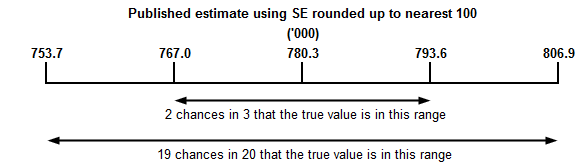PROPORTIONS AND PERCENTAGES 9 Proportions and percentages formed from the ratio of two estimates are also subject to sampling errors. The size of the error depends on the accuracy of both the numerator and the denominator. A formula to approximate the RSEs of proportions not provided in the spreadsheets is given below. This formula is only valid when x is a subset of y.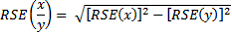10 Considering Datacube 3, of the 780,300 one parent families with a single wife/partner, 393,500 or (50.4%) were employed. The RSE of 393,500 is 3.3% and the RSE for 780,300 is 1.7%. Applying the above formula, the RSE for the proportion of persons who were employed: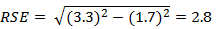11 Therefore, the SE for the proportion of single wife/partners who were employed was 1.4 percentage points (= (50.4/100) x 2.8). Therefore, there are about two chances in three that the proportion of one parent families with a single wife/partner who were employed is between 49.0% and 51.9%, and 19 chances in 20 that the proportion was within the range 47.6% to 53.3%. SUMS OR DIFFERENCES BETWEEN ESTIMATES 12 Published estimates may also be used to calculate the sum of two or more estimates, or the difference between two survey estimates (of numbers, means or percentages) where these are not provided in the spreadsheets. Such estimates are also subject to sampling error. 13 The sampling error of the difference between two estimates depends on their SEs and the relationship (correlation) between them. An approximate SE of the difference between two estimates (x–y) may be calculated by the following formula: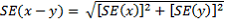14 The sampling error of the sum of two estimates is calculated in a similar way. An approximate SE of the sum of two estimates (x+y) may be calculated by the following formula: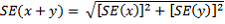15 Considering the example in paragraph 7, the estimated number of one parent families with a single wife/parent was 780,300, and the SE for this estimate was 13,300. From Datacube 3, the estimate of single mothers who were unemployed was 41,100 and the SE was 3,800. The estimate of single mothers who were unemployed: 780,300 + 41,100 = 821,400 16 The SE of the estimate of persons who were unemployed is: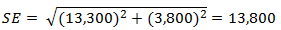17 Therefore, there are about two chances in three that the value that would have been produced if all households had been included in the survey would fall within the range 807,600 to 835,200 and about 19 chances in 20 that the value would fall within the range 793,700 to 849,000. 18 While these formulae will only be exact for sums of, or differences between, separate and uncorrelated characteristics or subpopulations, it is expected to provide a good approximation for all sums or differences likely to be of interest in this publication. SIGNIFICANCE TESTING 19 A statistical test for any comparisons between estimates can be performed to determine whether it is likely that there is a significant difference between two corresponding population characteristics. The standard error of the difference between two corresponding estimates (x and y) can be calculated using the formula in paragraph 9. This standard error is then used to calculate the following test statistic: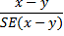20 If the value of this test statistic is greater than 1.96 then there is evidence, with a 95% level of confidence, of a statistically significant difference in the two populations with respect to that characteristic. Otherwise, it cannot be stated with confidence that there is a difference between the populations with respect to that characteristic. 21 The imprecision due to sampling variability, which is measured by the SE, should not be confused with inaccuracies that may occur because of imperfections in reporting by respondents and recording by interviewers, and errors made in coding and processing data. Inaccuracies of this kind are referred to as non-sampling error, and they occur in any enumeration, whether it be a full count or sample. Every effort is made to reduce non-sampling error to a minimum by careful design of questionnaires, intensive training and supervision of interviewers, and efficient operating procedures. Document Selection These documents will be presented in a new window.